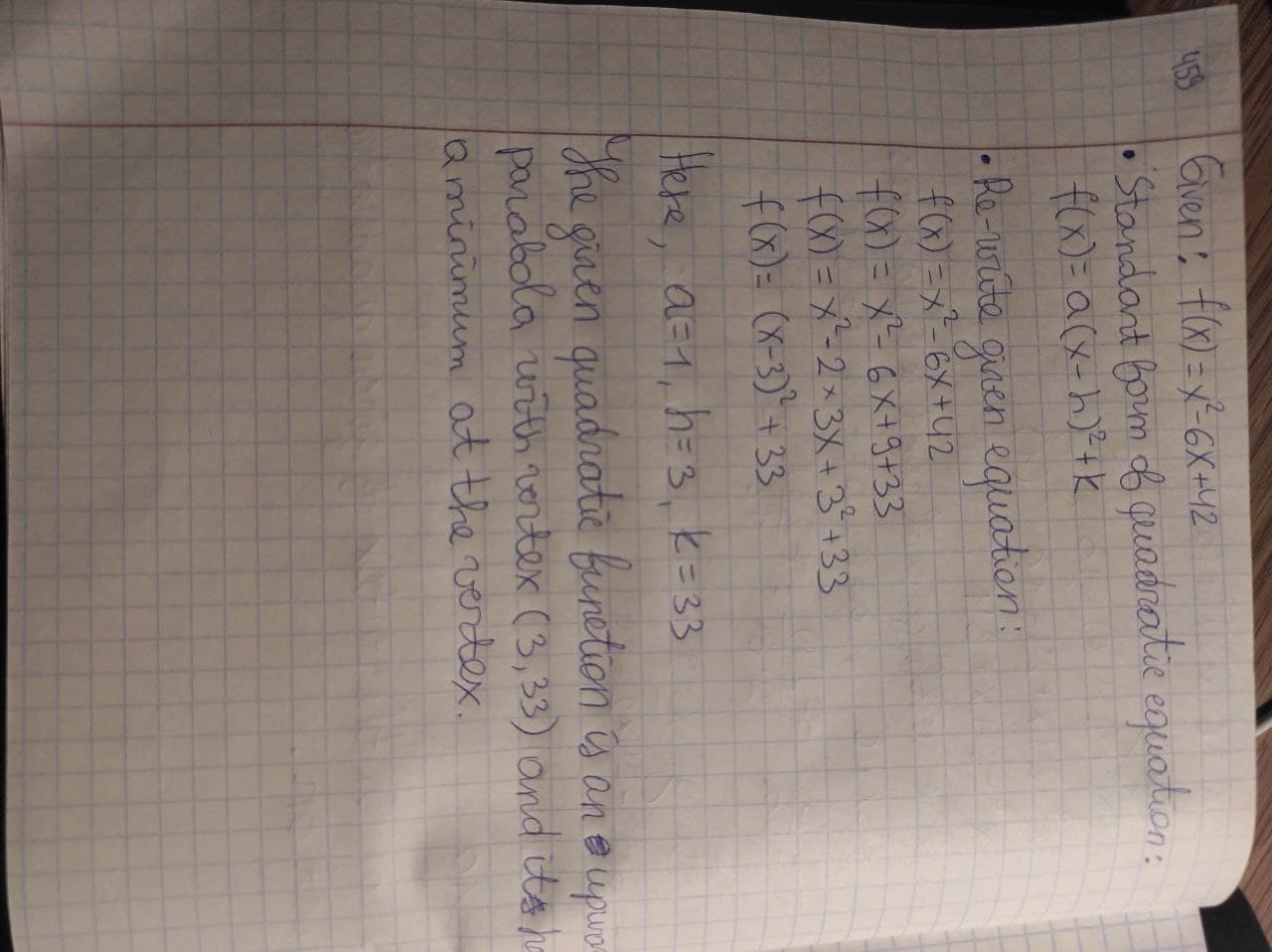# Find the vertex of the qudratic function f(x)=x^2-6x+42, then express the qudratic function in standart form f(x)=a(x-h)^2+k and state whether the vertex is a minimum or maximum. Enter exat answers only, no approximations.kuCAu 2021-03-01 Answered
Find the vertex of the qudratic function $$\displaystyle{f{{\left({x}\right)}}}={x}^{{2}}-{6}{x}+{42}$$, then express the qudratic function in standart form $$\displaystyle{f{{\left({x}\right)}}}={a}{\left({x}-{h}\right)}^{{2}}+{k}$$ and state whether the vertex is a minimum or maximum. Enter exat answers only, no approximations.

• Questions are typically answered in as fast as 30 minutes

### Plainmath recommends

• Get a detailed answer even on the hardest topics.
• Ask an expert for a step-by-step guidance to learn to do it yourself.curwyrm

To express the given quadratic function in standard form $$\displaystyle{f{{\left({x}\right)}}}={a}{\left({x}−{h}\right)}^{{2}}+{k}$$
Re-write the given quadratic function as:
$$\displaystyle{f{{\left({x}\right)}}}={x}^{{2}}−{6}{x}+{42}$$
$$\displaystyle{f{{\left({x}\right)}}}={x}^{{2}}−{6}{x}+{9}+{33}$$
$$\displaystyle{f{{\left({x}\right)}}}={x}^{{2}}−{2}×{3}{x}+{3}^{{2}}+{33}$$
$$\displaystyle{f{{\left({x}\right)}}}={\left({x}−{3}\right)}^{{2}}+{33}$$
The standard form of a given quadratic function is $$\displaystyle{f{{\left({x}\right)}}}={\left({x}−{3}\right)}^{{2}}+{33}$$
Here
$$a=1, h=3,$$ and $$k=33.$$
The given quadratic function is an upward parabola with vertex (3, 33) and it has a minimum at the vertex.